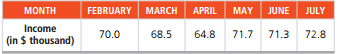Create an Account

Home / Questions / Resolve Problem 4.19 with a = .1 and b = .8. Using MSE, determine which smoothing constants..

Resolve Problem 4.19 with a = .1 and b = .8. Using MSE, determine which smoothing constants..

Resolve Problem 4.19 with a = .1 and b = .8. Using MSE, determine which smoothing constants provide a better forecast.

Problem 4.19

Income at the architectural firm Spraggins and Yunes for the period February to July was as follows:Use trend-adjusted exponential smoothing to forecast the firm’s August income. Assume that the initial forecast average for February is \$65,000 and the initial trend adjustment is 0. The smoothing constants selected are a = .1 and β = .2.

Jul 31 2020 View more View LessSubscribe To Get Solution